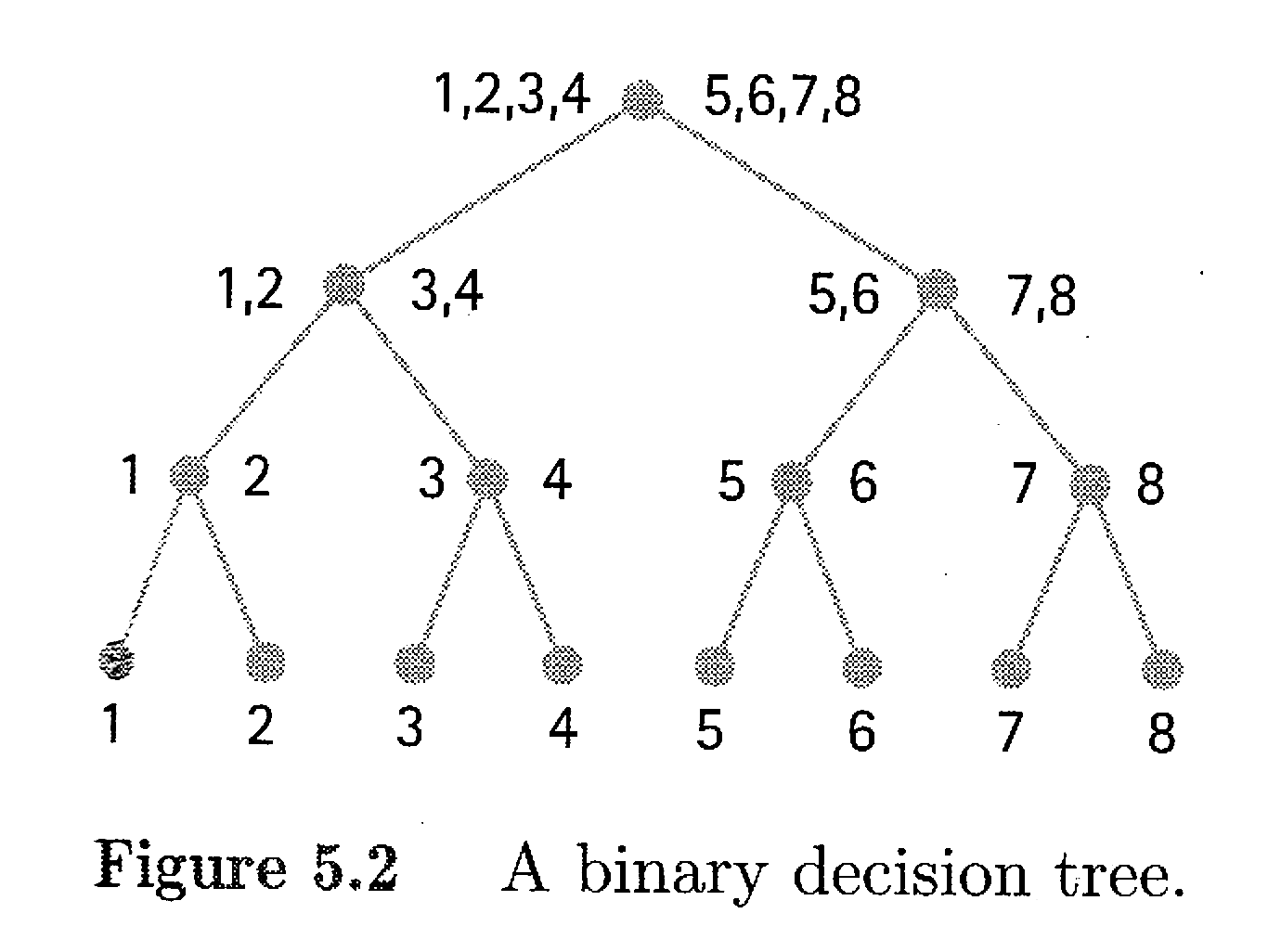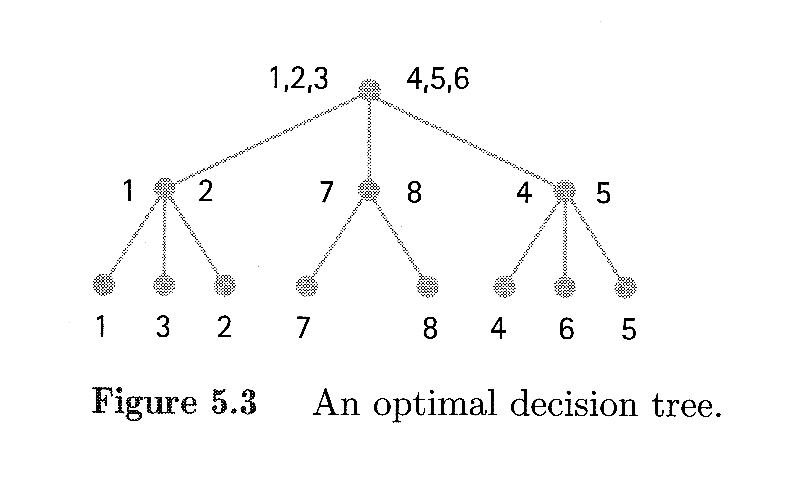# A Search Algorithm Puzzle: Finding a Bad Coin

By Alex Beal
August 17, 2011

Today I have a puzzle for you from James Hein’s textbook, Discrete Structures, Logic, and Computability. It’s a question about finding a “bad coin” and goes as follows:

Suppose we are asked to use a pan balance to find the heavy coin among eight coins with the assumption that they all look alike and the other seven all have the same weight. (p. 287) So, the problem is that we have 8 coins, and one of them is a bad coin that weighs more than the others. With only a pan balance, what’s the most efficient way to find the bad coin?

The simplest solution is to just weigh the coins in pairs. Grab a pair of coins and put one coin on each side of the balance. If one is heavier, you’ve found the bad coin and you can stop. If they weigh the same, move on to the next pair. At most, this will take 4 trials, but is this optimal? No. As James Hein points out, we can do better.

The better solution is to put 4 of the coins on one side of the balance, and the other 4 coins on the other side of the balance. One side must be heavier than the other, because one side must contain the heavier coin. If the left side is heavier, then we discard the lighter coins on the right side. We then split the remaining coins into 2 groups of 2, and repeat the procedure by weighing one group against the other. The heavier of the two pairs then gets split and weighed. The coin that is heavier is the bad coin. This is a sure way to find the bad coin in 3 steps.

Programmers will recognize this algorithm as a binary search, which can be represented by the following decision tree from page 287 of Hein’s book:First we weigh 1 through 4 against 5 through 8. If 1 through 4 is heavier, then we weigh 1 and 2 against 3 and 4 and so on until we reach the bottom of the tree. It’s a neat solution that has lots of uses. The obvious application for this is searching through lots of data, but an especially cool use is the Huffman coding algorithm. A variation on Huffman coding is how zip archives are compressed.

But wait a minute. We can do one better by allowing some decision points (nodes) on the tree to have three connections rather than two, making it a ternary tree. See the revised, and optimal, decision tree below (Hein, p. 288):For this revised procedure, we begin by weighing 1 through 3 and 4 through 6. If those groups have equal weight, then we go down the middle branch and see that the bad coin must be 7 or 8. We follow a similar procedure if, after the first weighing, 1 through 3 is heavier. We go down the left branch and test 1 and 2. If those weigh the same, then the bad coin must be coin 3. Pretty clever.

Next post I’ll attempt some mathematical analysis on the algorithms, and see what we can say about how many steps it takes to sort through n coins. In the meantime, check out this interesting tidbit from Wikipedia’s Binary Search article. Despite its ubiquity and seeming simplicity, it’s apparently quite difficult to implement!